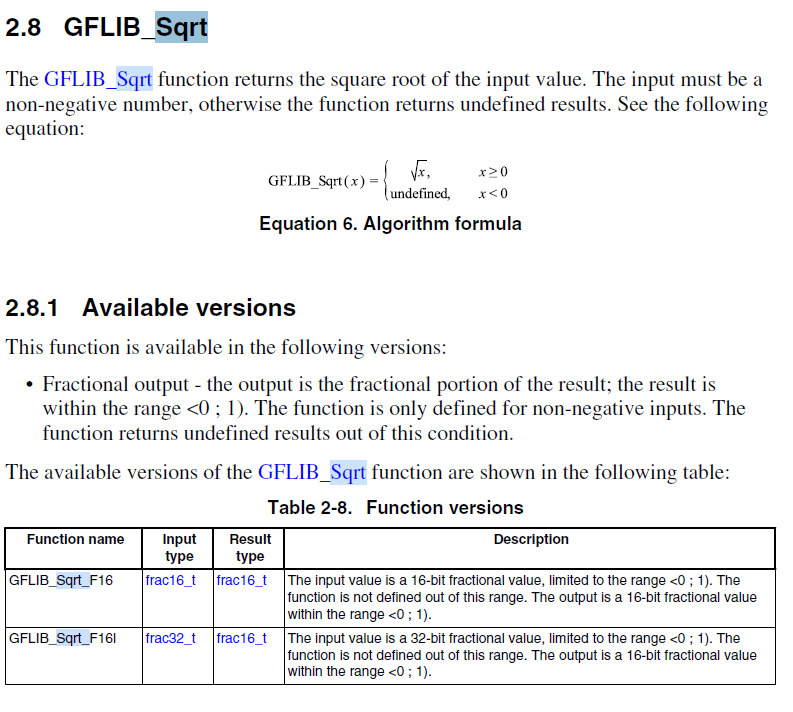## DSP_Func_MFR library

cancel
Showing results for
Did you mean:

## DSP_Func_MFR library

63 Views
Contributor I

The chip I used is 56F84786, I want to use the DSP_Func_MFR library inside, and use MFR_mfr32Sqrt to calculate the square root, the returned results are all wrong, may I ask whether other configuration is needed?

6 ViewsNXP TechSupport

Hi, ChenJian,

First of all, I think the DSP_Func_MFR is very old, I suggest that you use the latest library RTCESL which adapts to DSP56800EX core.

You can use the GFLIB_sqrt_F16() function to calculate the value.

Note no matter whether you use GFLIB_sqrt_F16() or MFR_mfr32Sqrt (), the input value variable format is Frac16 or Frac32. For example, for 16 bits Frac16 variable:

Frac16_t var=0x4000;  //the var value is 0.5

Frac16_t res=GFLIB_sqrt_F16(var) ; //res variable should be 0.7071*32768=23170 in decimal